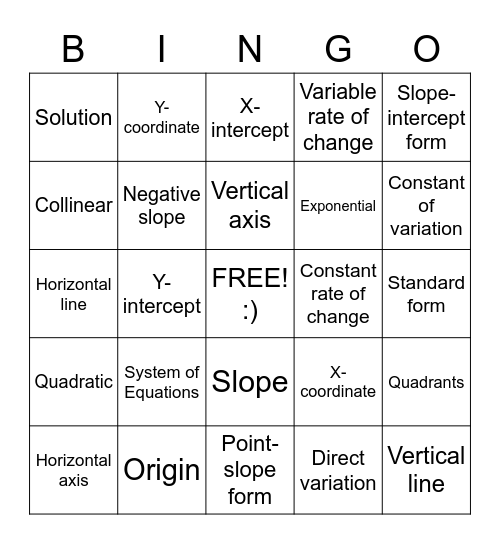# EquationsThis bingo card has a free space and 30 words: Origin, Parabola, Y-intercept, Undefined slope, Solution, X-intercept, Negative slope, Slope, Constant rate of change, Point-slope form, Standard form, Vertical line, Direct variation, Positive slope, Constant of variation, Zero slope, X-coordinate, Quadratic, System of Equations, Slope-intercept form, Horizontal line, Ordered pair, Y-coordinate, Exponential, Collinear, Variable rate of change, Quadrants, Horizontal axis, Vertical axis and Coordinate Plane.

## Play Online